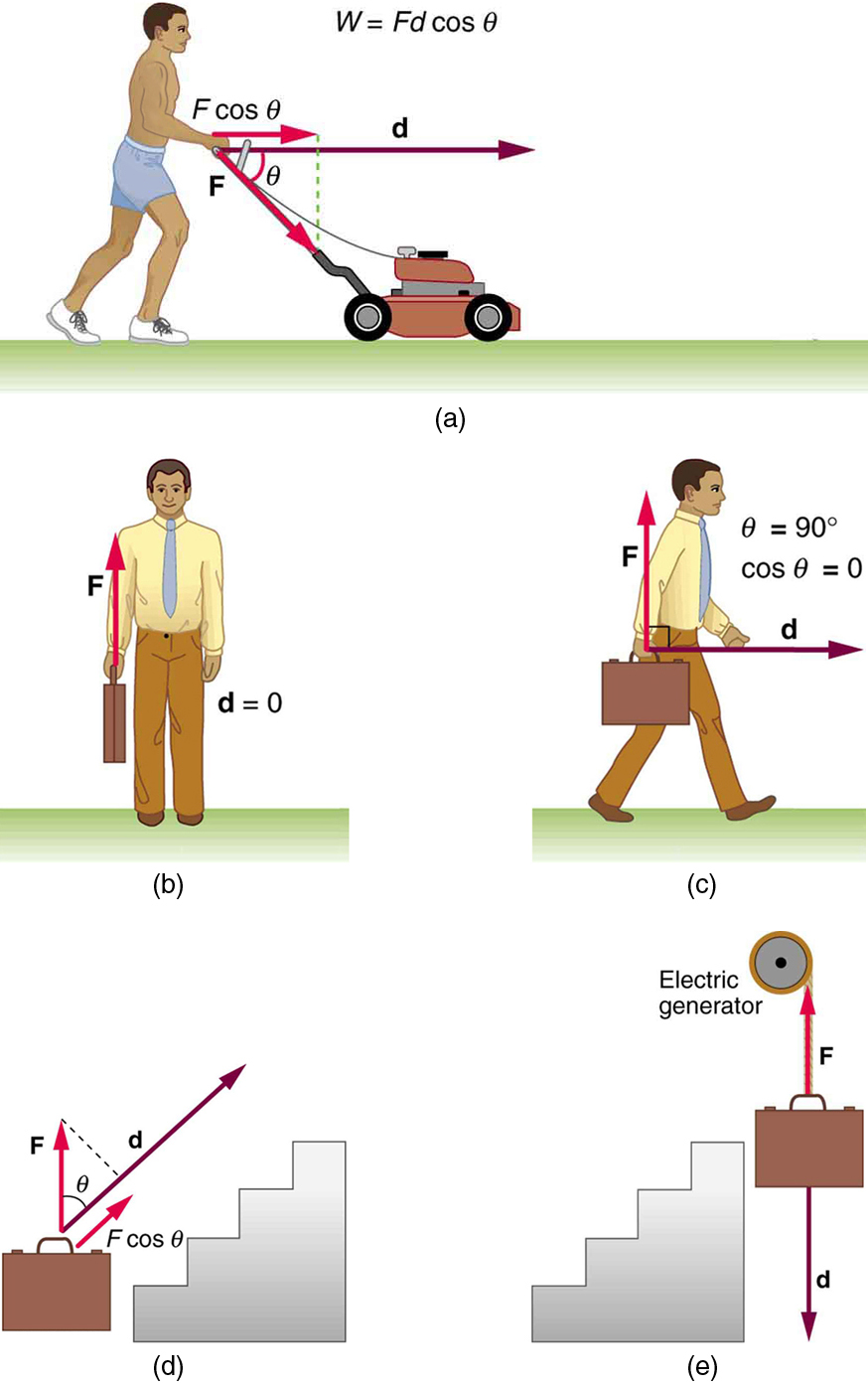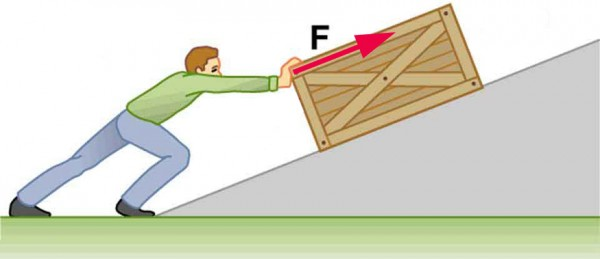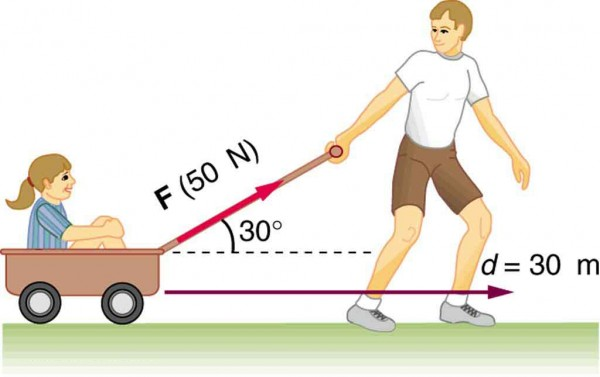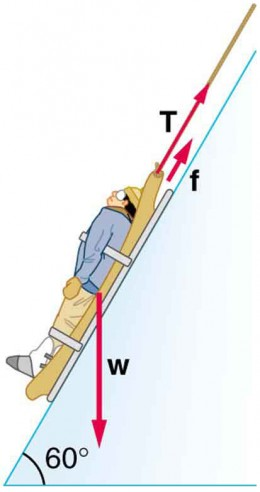## Work: The Scientific Definition

### Learning Objectives

By the end of this section, you will be able to:

• Explain how an object must be displaced for a force on it to do work.
• Explain how relative directions of force and displacement determine whether the work done is positive, negative, or zero.

## What It Means to Do Work

The scientific definition of work differs in some ways from its everyday meaning. Certain things we think of as hard work, such as writing an exam or carrying a heavy load on level ground, are not work as defined by a scientist. The scientific definition of work reveals its relationship to energy—whenever work is done, energy is transferred.

For work, in the scientific sense, to be done, a force must be exerted and there must be motion or displacement in the direction of the force.

Formally, the work done on a system by a constant force is defined to be the product of the component of the force in the direction of motion times the distance through which the force acts. For one-way motion in one dimension, this is expressed in equation form as W = |F| (cosθ) |d|, where W is work, d is the displacement of the system, and θ is the angle between the force vector F and the displacement vector d, as in Figure 1. We can also write this as WFd cos θ.

To find the work done on a system that undergoes motion that is not one-way or that is in two or three dimensions, we divide the motion into one-way one-dimensional segments and add up the work done over each segment.

### What is Work?

The work done on a system by a constant force is the product of the component of the force in the direction of motion times the distance through which the force acts. For one-way motion in one dimension, this is expressed in equation form as WFd cos θ, where W is work, F is the magnitude of the force on the system, d is the magnitude of the displacement of the system, and θ is the angle between the force vector F and the displacement vector d.Figure 1. examples of work. (a) The work done by the force F on this lawn mower is Fd cos θ. Note that F cos θ is the component of the force in the direction of motion. (b) A person holding a briefcase does no work on it, because there is no motion. No energy is transferred to or from the briefcase. (c) The person moving the briefcase horizontally at a constant speed does no work on it, and transfers no energy to it. (d) Work is done on the briefcase by carrying it up stairs at constant speed, because there is necessarily a component of force F in the direction of the motion. Energy is transferred to the briefcase and could in turn be used to do work. (e) When the briefcase is lowered, energy is transferred out of the briefcase and into an electric generator. Here the work done on the briefcase by the generator is negative, removing energy from the briefcase, because F and d are in opposite directions.

To examine what the definition of work means, let us consider the other situations shown in Figure 1. The person holding the briefcase in Figure 1b does no work, for example. Here d = 0, so W = 0. Why is it you get tired just holding a load? The answer is that your muscles are doing work against one another, but they are doing no work on the system of interest (the “briefcase-Earth system”—see Gravitational Potential Energy for more details). There must be motion for work to be done, and there must be a component of the force in the direction of the motion. For example, the person carrying the briefcase on level ground in Figure 1c does no work on it, because the force is perpendicular to the motion. That is, cos 90º = 0, and so W = 0.

In contrast, when a force exerted on the system has a component in the direction of motion, such as in Figure 1d, work is done—energy is transferred to the briefcase. Finally, in Figure 1e, energy is transferred from the briefcase to a generator. There are two good ways to interpret this energy transfer. One interpretation is that the briefcase’s weight does work on the generator, giving it energy. The other interpretation is that the generator does negative work on the briefcase, thus removing energy from it. The drawing shows the latter, with the force from the generator upward on the briefcase, and the displacement downward. This makes θ = 180º, and cos 180º = −1; therefore, W is negative.

## Calculating Work

Work and energy have the same units. From the definition of work, we see that those units are force times distance. Thus, in SI units, work and energy are measured in newton-meters. A newton-meter is given the special name joule (J), and 1J = 1N · m = 1 kg · m2/s2. One joule is not a large amount of energy; it would lift a small 100-gram apple a distance of about 1 meter.

### Example 1. Calculating the Work You Do to Push a Lawn Mower Across a Large Lawn

How much work is done on the lawn mower by the person in Figure 1a if he exerts a constant force of 75.0 N at an angle 35º below the horizontal and pushes the mower 25.0 m on level ground? Convert the amount of work from joules to kilocalories and compare it with this person’s average daily intake of 10,000 kJ (about 2400 kcal) of food energy. One calorie (1 cal) of heat is the amount required to warm 1 g of water by 1ºC, and is equivalent to 4.184 J, while one food calorie (1 kcal) is equivalent to 4184 J.

#### Strategy

We can solve this problem by substituting the given values into the definition of work done on a system, stated in the equation WFd cos θ. The force, angle, and displacement are given, so that only the work W is unknown.

#### Solution

The equation for the work is WFd cos θ.

Substituting the known values gives

$\begin{array}{lll}W&=&(75.0\text{ N})(25.0\text{ m})\cos(35.0^{\circ})\\\text{ }&=&1536\text{ J}=1.54\times10^3\text{ J}\end{array}\\$

Converting the work in joules to kilocalories yields W = (1536 J)(1 kcal/4184 J) = 0.367 kcal. The ratio of the work done to the daily consumption is

$\displaystyle\frac{W}{2400\text{ kcal}}=1.53\times10^{-4}\\$

#### Discussion

This ratio is a tiny fraction of what the person consumes, but it is typical. Very little of the energy released in the consumption of food is used to do work. Even when we “work” all day long, less than 10% of our food energy intake is used to do work and more than 90% is converted to thermal energy or stored as chemical energy in fat.

## Section Summary

Work is the transfer of energy by a force acting on an object as it is displaced.

The work W that a force F does on an object is the product of the magnitude F of the force, times the magnitude d of the displacement, times the cosine of the angle θ between them. In symbols, WFd cos θ.

The SI unit for work and energy is the joule (J), where 1 J = 1 N ⋅ m = 1 kg ⋅ m2/s2.

The work done by a force is zero if the displacement is either zero or perpendicular to the force.

The work done is positive if the force and displacement have the same direction, and negative if they have opposite direction.

### Conceptual Questions

1. Give an example of something we think of as work in everyday circumstances that is not work in the scientific sense. Is energy transferred or changed in form in your example? If so, explain how this is accomplished without doing work.
2. Give an example of a situation in which there is a force and a displacement, but the force does no work. Explain why it does no work.
3. Describe a situation in which a force is exerted for a long time but does no work. Explain.

### Problems & Exercises

1. How much work does a supermarket checkout attendant do on a can of soup he pushes 0.600 m horizontally with a force of 5.00 N? Express your answer in joules and kilocalories.
2. A 75.0-kg person climbs stairs, gaining 2.50 meters in height. Find the work done to accomplish this task.
3. (a) Calculate the work done on a 1500-kg elevator car by its cable to lift it 40.0 m at constant speed, assuming friction averages 100 N. (b) What is the work done on the lift by the gravitational force in this process? (c) What is the total work done on the lift?
4. Suppose a car travels 108 km at a speed of 30.0 m/s, and uses 2.0 gal of gasoline. Only 30% of the gasoline goes into useful work by the force that keeps the car moving at constant speed despite friction. (A gallon of gasoline has 1.2 × 108 J.) (a) What is the magnitude of the force exerted to keep the car moving at constant speed? (b) If the required force is directly proportional to speed, how many gallons will be used to drive 108 km at a speed of 28.0 m/s?
5. Calculate the work done by an 85.0-kg man who pushes a crate 4.00 m up along a ramp that makes an angle of 20.0º with the horizontal. (See Figure 2.) He exerts a force of 500 N on the crate parallel to the ramp and moves at a constant speed. Be certain to include the work he does on the crate and on his body to get up the ramp.Figure 2. A man pushes a crate up a ramp.

6. How much work is done by the boy pulling his sister 30.0 m in a wagon as shown in Figure 3? Assume no friction acts on the wagon.Figure 3. The boy does work on the system of the wagon and the child when he pulls them as shown.

7. A shopper pushes a grocery cart 20.0 m at constant speed on level ground, against a 35.0 N frictional force. He pushes in a direction 25.0º below the horizontal. (a) What is the work done on the cart by friction? (b) What is the work done on the cart by the gravitational force? (c) What is the work done on the cart by the shopper? (d) Find the force the shopper exerts, using energy considerations. (e) What is the total work done on the cart?
8. Suppose the ski patrol lowers a rescue sled and victim, having a total mass of 90.0 kg, down a 60.0º slope at constant speed, as shown in Figure 4. The coefficient of friction between the sled and the snow is 0.100. (a) How much work is done by friction as the sled moves 30.0 m along the hill? (b) How much work is done by the rope on the sled in this distance? (c) What is the work done by the gravitational force on the sled? (d) What is the total work done?Figure 4. A rescue sled and victim are lowered down a steep slope.

## Glossary

energy: the ability to do work

work: the transfer of energy by a force that causes an object to be displaced; the product of the component of the force in the direction of the displacement and the magnitude of the displacement

joule: SI unit of work and energy, equal to one newton-meter

### Selected Solutions to Problems & Exercises

1. 3.00 J = 7.17 × 10−4 kcal

3. (a) 5.92 × 105 J; (b) −5.88 × 105 J; (c) The net force is zero.

5. 3.14 × 103 J

7.  (a) −700 J; (b) 0; (c) 700 J; (d) 38.6 N; (e) 0